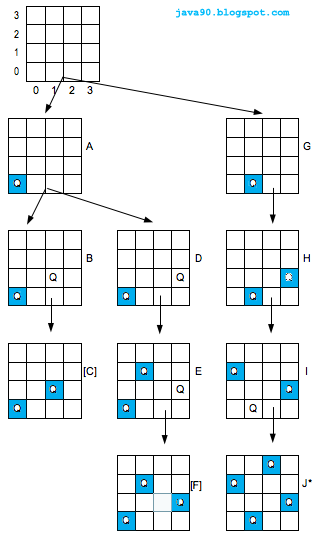# The n-queens Problem in Java

A classic combinatorial problem is to place n queens on an n × n chess board so that no queen threatens any other queen, that is, so that no two queens are on the same row, column, or diagonal. In order to reduce this solution space, it can now be assumed without loss of generality, that the ith queen is placed in the ith row, thereby avoiding those two queens can be in the same row.

All solutions to the n-queens problem can therefore be represented as ntuples (c1, c2, . . . cn), where ci is column on which queen i is placed, and no two ciscan be the same. Each queen is assigned a different column, so the only thing left to check is whether two queens are in the same diagonal. The rows are now numbered from bottom to top and the columns are numbered from left to right Hence the bottom left corner has the coordinates (0,0) and the top left corner (n-1,0). With this numeration, two queens with the coordinates (i, ci) and (j, cj) are in the same ascending diagonal if j −i == cjci. They are in the same descending diagonal if j −i == ci − cj. Thus, in order to check whether two queens are in the same diagonal, no matter in which direction, it is sufficient to check whether |j − i| == |cj − ci| or not.

### Algorithm

We will use Backtracking algorithm for placing N Queens on N*N chess board.

1. Since Queens attack on same rows, so only one Queen per row can be set.
2. Since Queens attack on same column, so only one Queen per column can be set.
3. We can start placing Queens either column wise that is one column at a time or can start placing . Queens row wise that is one row at a time. We will be using Row wise placement. Start from Row 0, check the safe position to place on Row 0 and place a queen on row 0. once queen is placed on Row 0, move to Row 1 and so on.
4. Before placing a queen on any position in a row, we first check is there any other already placed queens on prior rows attacking on this position.if no already placed queen is attacking, then only place a queen on position.
5. So when we are working on say Row 2. and we are looking for safe position on Row 2,then we need to check the safe position on Row 2 against Row 1 and Row 0 as that are the rows where we already placed a Queens.
6. If queen placement is not leading to a solution, then backtrack and change the position of already placed queen and retry.* Q * *
* * * Q
Q * * *
* * Q *

* * Q *
Q * * *
* * * Q
* Q * *